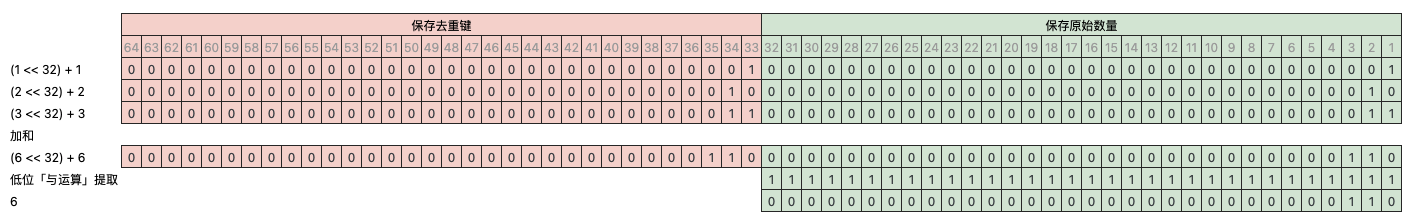## 如何做去重加和

• 键鼠套装组合销售，组合了一个键盘两个鼠标，商品 ID 为 123，键盘鼠标分别对应库存系统里的两个 SKU JP1 和 SB1
• 键盘 JP1 也单独销售，商品 ID 为 456，
• 同样鼠标 SB1 也单独销售，商品 ID 为 789

• 订单 o1 买了 1 个 123 键鼠套装
• 订单 o2 买了 2 个 456 键盘
• 订单 o3 买了 1 个 789 鼠标

``````CREATE TABLE f_order
(
`orderID` String,
`productID` UInt32,
`sku` String,
`orgQuantity` UInt16,
`realQuantity` UInt16
)
ENGINE = MergeTree
ORDER BY orderID

INSERT INTO f_order VALUES ('o1', 123, 'JP1', 1, 1), ('o1', 123, 'SB1', 1, 2), ('o2', 456, 'JP1', 2, 2), ('o3', 789, 'SB1', 1, 1)

SELECT *
FROM f_order

┌─orderID─┬─productID─┬─sku─┬─orgQuantity─┬─realQuantity─┐
│ o1      │       123 │ JP1 │           1 │            1 │
│ o1      │       123 │ SB1 │           1 │            2 │
│ o2      │       456 │ JP1 │           2 │            2 │
│ o3      │       789 │ SB1 │           1 │            1 │
└─────────┴───────────┴─────┴─────────────┴──────────────┘
``````

orgQurantity 表示原始销售数量
realQuantity 表示库存实际扣减的数量

• 统计原始销量汇总为 4（123：1，456：2，789：1）
• 统计商品（productID）维度的原始销量为 123：1，456：2，789：1
• 统计 sku 维度的实际销量为 JP1：3，SB1：3
• 统计 sku 维度的原始销量为 JP1：3，SB1：2
• ...

### 常规做法

``````SELECT sum(orgQuantity) AS totalOrgQuantity
FROM
(
SELECT any(orgQuantity) AS orgQuantity
FROM f_order
GROUP BY orderID, productID
)

┌─totalOrgQuantity─┐
│                4 │
└──────────────────┘
``````

### ClickHouse 做法

#### 做法一

``````SELECT arraySum(x -> (x.3), groupUniqArray((orderID, productID, orgQuantity))) AS totalOrgQuantity
FROM f_order

┌─totalOrgQuantity─┐
│                4 │
└──────────────────┘
``````

``````SELECT sum(number) AS x
FROM
(
SELECT number
FROM numbers(10000000)
)

┌──────────────x─┐
│ 49999995000000 │
└────────────────┘

1 rows in set. Elapsed: 0.012 sec. Processed 10.02 million rows, 80.18 MB (814.14 million rows/s., 6.51 GB/s.)

SELECT arraySum(x -> x, groupUniqArray(number)) AS x
FROM numbers(10000000)

┌──────────────x─┐
│ 49999995000000 │
└────────────────┘

1 rows in set. Elapsed: 1.264 sec. Processed 10.02 million rows, 80.18 MB (7.93 million rows/s., 63.45 MB/s.)
``````

#### 做法二

``````-- 将 orgQuantity 修改为 Tuple(k Nullable(UInt32), q UInt16) 类型
-- 第一位 k 作去重键，取值 NULL 表示非组合商品，有值时为组合商品当前订单的订单号和商品 ID 的 crc32 值
CREATE TABLE f_order_2
(
`orderID` String,
`productID` UInt32,
`sku` String,
`orgQuantity` Tuple(k Nullable(UInt32), q UInt16),
`realQuantity` UInt16
)
ENGINE = MergeTree
ORDER BY orderID
SETTINGS index_granularity = 8192

-- 从 f_order 中导入数据，仅 productID 为 123 组合商品时才赋值给 orgQuantity.k
INSERT INTO f_order_2 SELECT
orderID,
productID,
sku,
(if(productID = 123, crc32(concat(orderID, toString(productID))), NULL), orgQuantity),
realQuantity
FROM f_order

SELECT *
FROM f_order_2

┌─orderID─┬─productID─┬─sku─┬─orgQuantity────┬─realQuantity─┐
│ o1      │       123 │ JP1 │ (3806212741,1) │            1 │
│ o1      │       123 │ SB1 │ (3806212741,1) │            2 │
│ o2      │       456 │ JP1 │ (NULL,2)       │            2 │
│ o3      │       789 │ SB1 │ (NULL,1)       │            1 │
└─────────┴───────────┴─────┴────────────────┴──────────────┘
``````

``````SELECT arraySum(x -> (x.2), groupUniqArrayIf(orgQuantity, isNotNull(orgQuantity.k))) + sumIf(orgQuantity.q, isNull(orgQuantity.k)) AS totalOrgQuantity
FROM f_order_3

┌─totalOrgQuantity─┐
│                4 │
└──────────────────┘
``````

#### 做法三``````-- 将 orgQuantity 修改为 UInt64 类型
-- 高位 33 ~ 64 用来保存「去重键」, 为 0 表示非组合商品，有值时为组合商品当前订单的订单号和商品 ID 的 crc32 值
CREATE TABLE f_order_3
(
`orderID` String,
`productID` UInt32,
`sku` String,
`orgQuantity` UInt64,
`realQuantity` UInt16
)
ENGINE = MergeTree
ORDER BY orderID
SETTINGS index_granularity = 8192

-- 从 f_order 中导入数据，仅 productID 为 123 组合商品时才赋值给 orgQuantity.k
INSERT INTO f_order_3 SELECT
orderID,
productID,
sku,
bitShiftLeft(toUInt64(if(productID = 123, crc32(concat(orderID, toString(productID))), 0)), 32) + orgQuantity,
realQuantity
FROM f_order

SELECT *
FROM f_order_3

┌─orderID─┬─productID─┬─sku─┬──────────orgQuantity─┬─realQuantity─┐
│ o1      │       123 │ JP1 │ 16347559244213518337 │            1 │
│ o1      │       123 │ SB1 │ 16347559244213518337 │            2 │
│ o2      │       456 │ JP1 │                    2 │            2 │
│ o3      │       789 │ SB1 │                    1 │            1 │
└─────────┴───────────┴─────┴──────────────────────┴──────────────┘
``````

``````-- 统计原始销量汇总为 4（123：1，456：2，789：1）
SELECT bitAnd(sumDistinctIf(orgQuantity, orgQuantity >= 4294967296), 4294967295) + sumIf(orgQuantity, orgQuantity < 4294967296) AS totalOrgQuantity
FROM f_order_3

┌─totalOrgQuantity─┐
│                4 │
└──────────────────┘

-- 统计商品（productID）维度的原始销量为 123：1，456：2，789：1
SELECT
productID,
bitAnd(sumDistinctIf(orgQuantity, orgQuantity >= 4294967296), 4294967295) + sumIf(orgQuantity, orgQuantity < 4294967296) AS totalOrgQuantity
FROM f_order_3
GROUP BY productID

┌─productID─┬─totalOrgQuantity─┐
│       456 │                2 │
│       789 │                1 │
│       123 │                1 │
└───────────┴──────────────────┘

-- 统计 sku 维度的实际销量为 JP1：3，SB1：3
SELECT
sku,
sum(realQuantity) AS totalRealQuantity
FROM f_order_3
GROUP BY sku

┌─sku─┬─totalRealQuantity─┐
│ JP1 │                 3 │
│ SB1 │                 3 │
└─────┴───────────────────┘

-- 统计 sku 维度的原始销量为 JP1：3，SB1：2
SELECT
sku,
bitAnd(sumDistinctIf(orgQuantity, orgQuantity >= 4294967296), 4294967295) + sumIf(orgQuantity, orgQuantity < 4294967296) AS totalOrgQuantity
FROM f_order_3
GROUP BY sku

┌─sku─┬─totalOrgQuantity─┐
│ JP1 │                3 │
│ SB1 │                2 │
└─────┴──────────────────┘
``````

#### 小结

``````CREATE TABLE x_tuple
(
`x` Tuple(Nullable(UInt32), UInt16)
)
ENGINE = MergeTree
ORDER BY x

-- 模拟 9 / 10 的数据为非组合商品（没有去重键）
INSERT INTO x_tuple SELECT x
FROM
(
SELECT
number % 100000 AS y,
(if(y > 90000, NULL, y), (number % 4) + 1) AS x
FROM numbers(10000000)
)

SELECT arraySum(x -> (x.2), groupUniqArrayIf(x, isNotNull(x.1))) + sumIf(x.2, isNull(x.1)) AS x
FROM x_tuple

┌───────x─┐
│ 2724901 │
└─────────┘

1 rows in set. Elapsed: 0.381 sec. Processed 10.00 million rows, 70.00 MB (26.26 million rows/s., 183.81 MB/s.)
``````

``````CREATE TABLE x_distinct
(
`x` UInt64
)
ENGINE = MergeTree
ORDER BY x

-- 模拟 9 / 10 的数据为非组合商品（没有去重键）
INSERT INTO x_distinct SELECT x
FROM
(
SELECT
number % 100000 AS y,
(if(y > 90000, 0, bitShiftLeft(toUInt64(y + 1), 32)) + (number % 4)) + 1 AS x
FROM numbers(10000000)
)

SELECT bitAnd(sumDistinctIf(x, x >= 4294967296), 4294967295) + sumIf(x, x < 4294967296) AS x
FROM x_distinct

┌───────x─┐
│ 2724901 │
└─────────┘

1 rows in set. Elapsed: 0.170 sec. Processed 10.00 million rows, 80.00 MB (58.71 million rows/s., 469.69 MB/s.)
``````